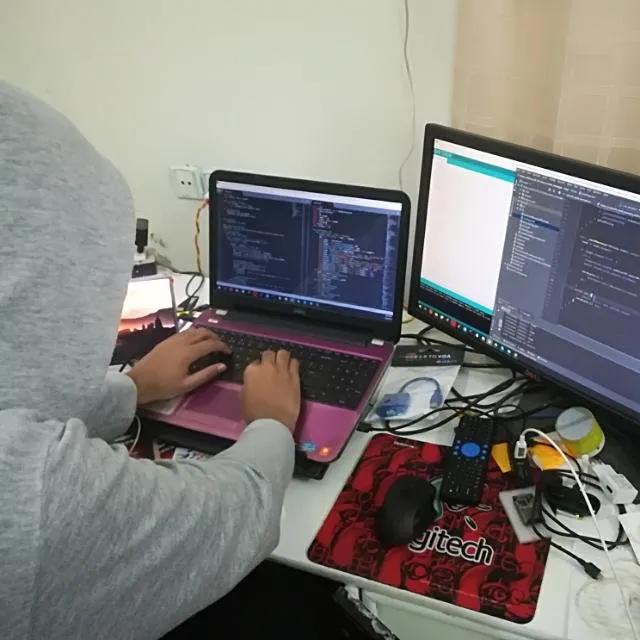Published

# Arduino Button Tutorial Using Arduino DigitalRead Function

In this tutorial, you are going to learn about interfacing the button with Arduino using the Arduino digitalRead function.

BeginnerProtip1 hour63,835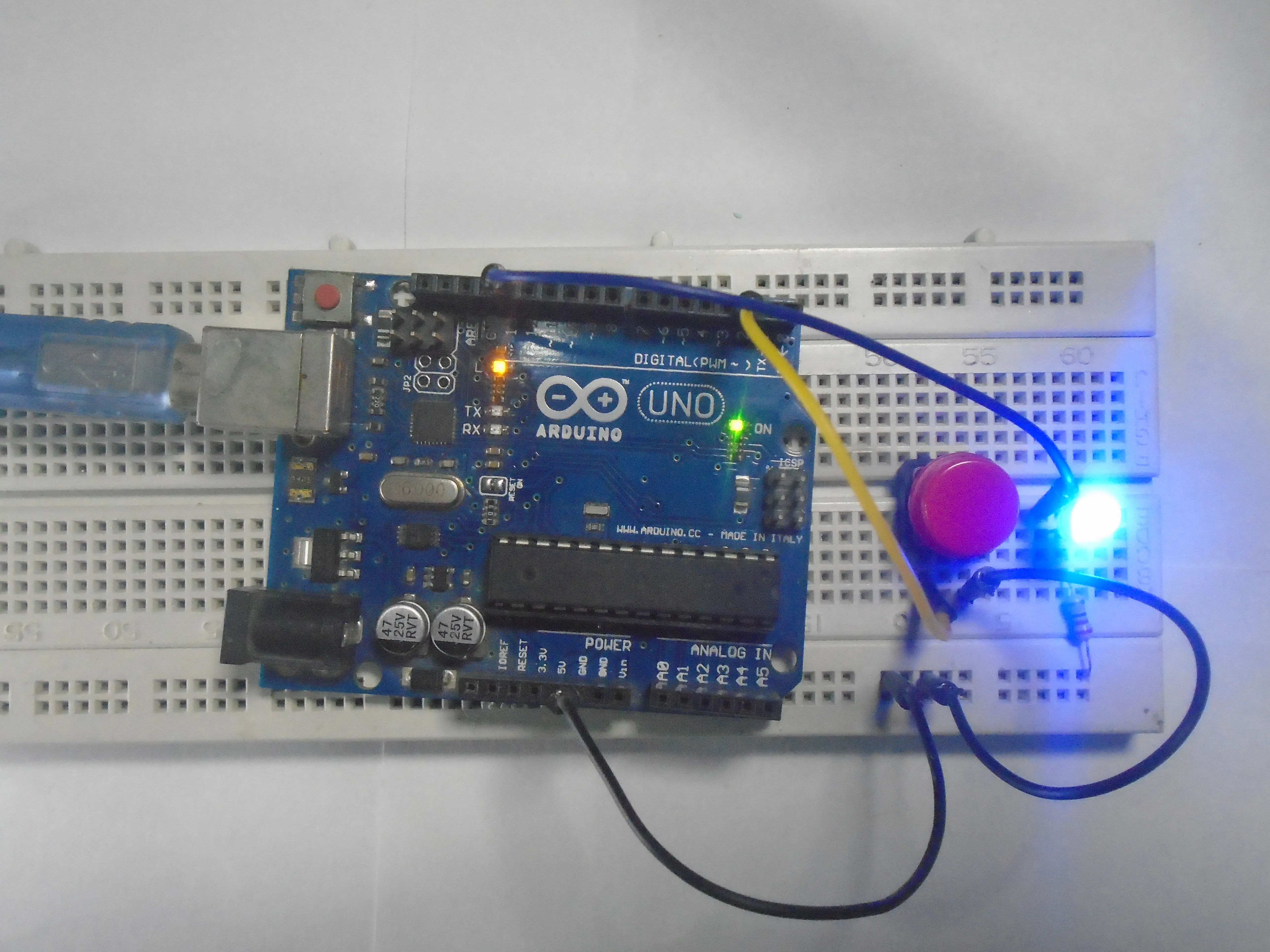## Things used in this project

### Hardware componentsArduino UNO & Genuino UNO
×1SparkFun Pushbutton switch 12mm
×15 mm LED: Red
×1Resistor 10k ohm
×1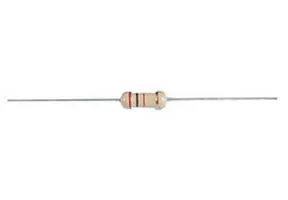Resistor 220 ohm
×1Jumper wires (generic)
×1×1

### Software apps and online servicesArduino IDE

## Schematics

### Circuit Diagram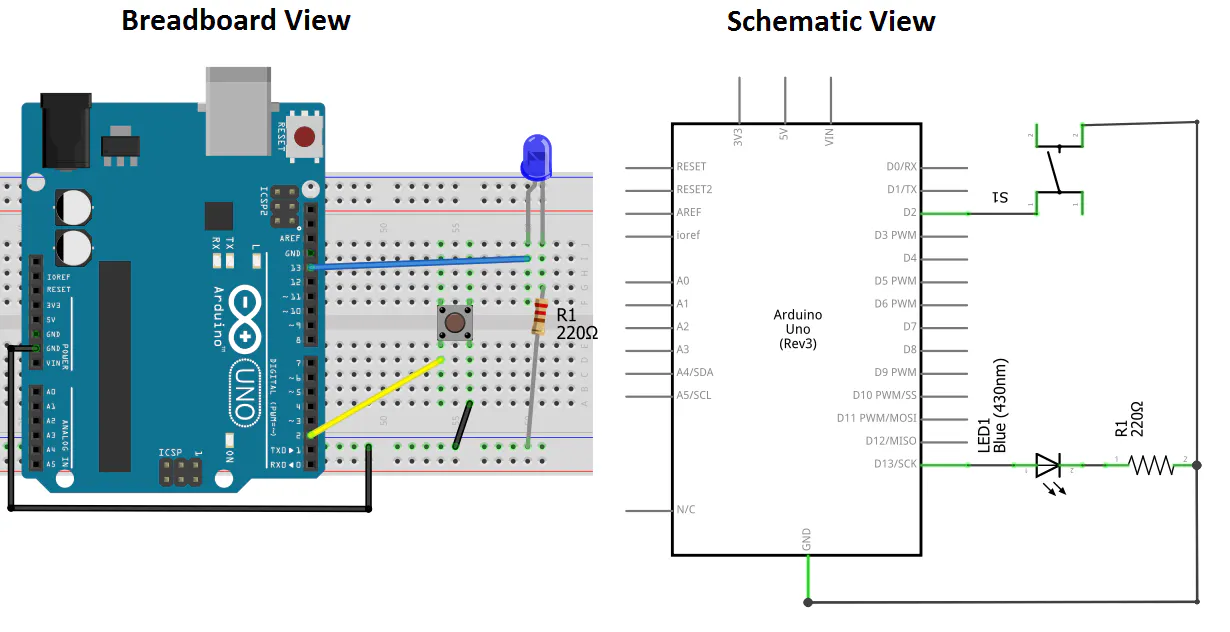### Circuit Diagram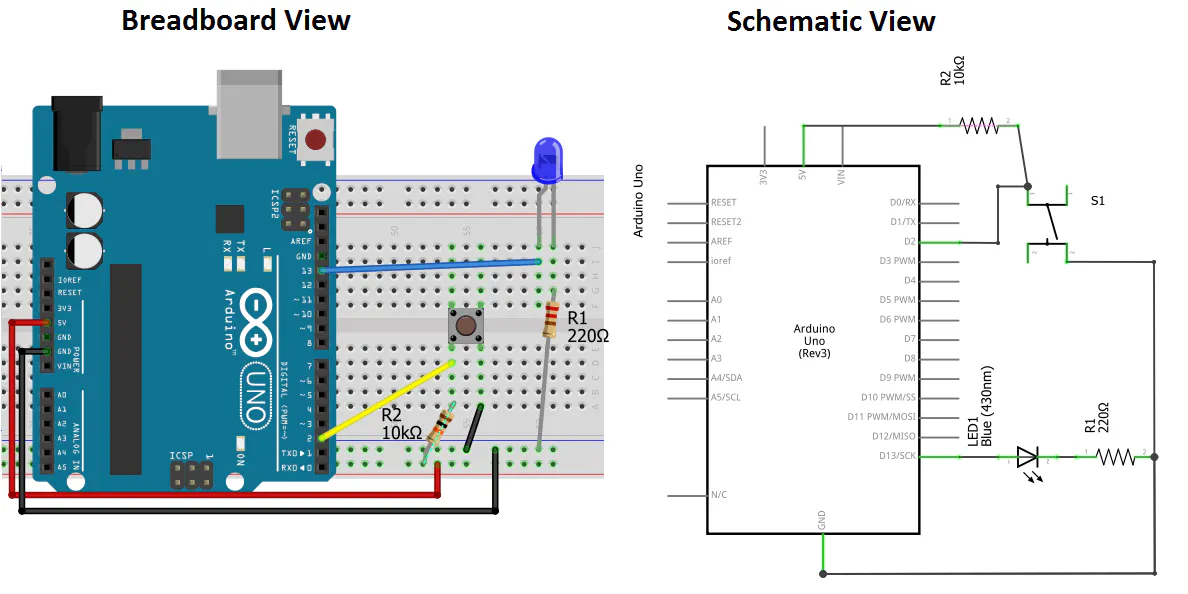### Circuit Diagram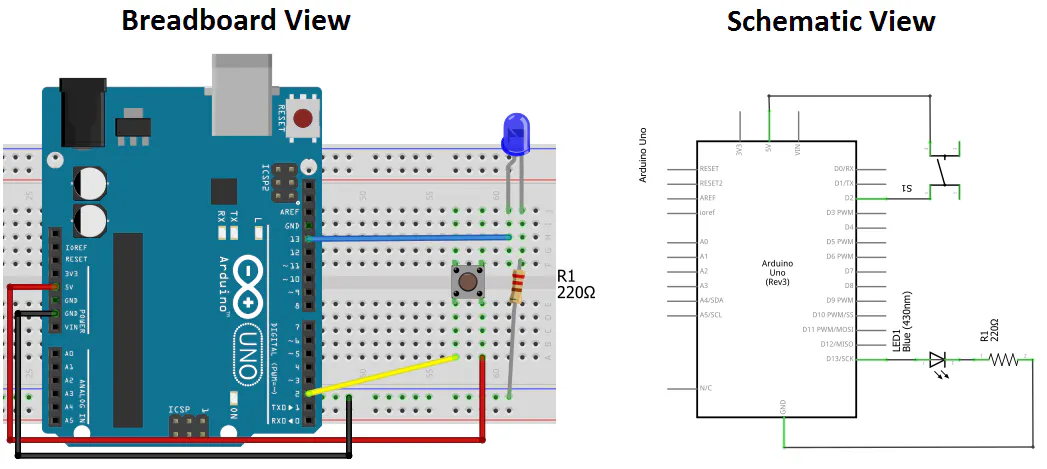### Circuit Diagram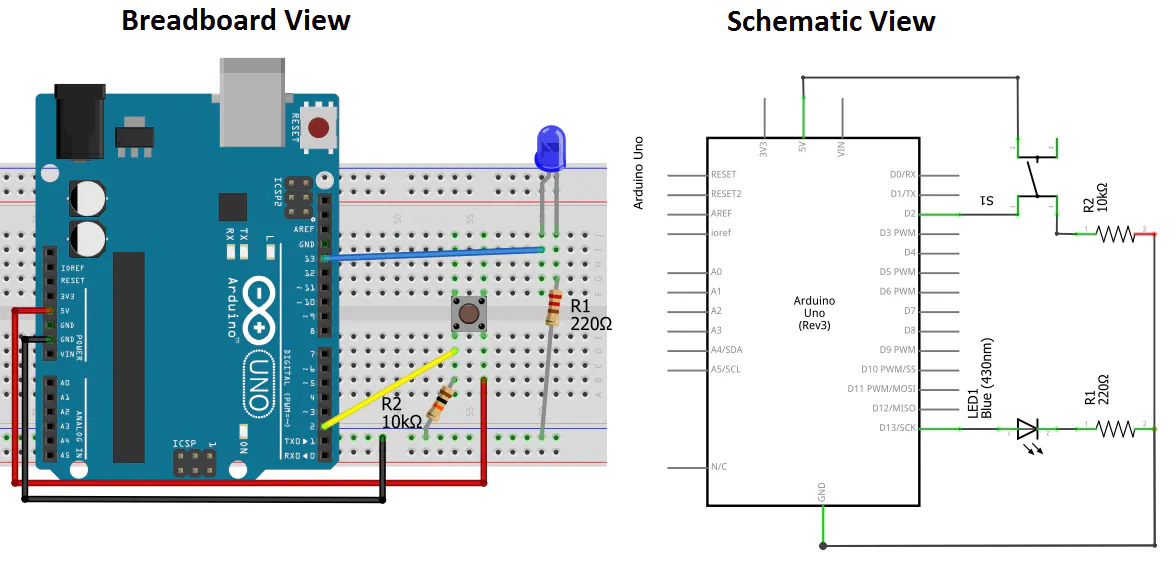## Code

### Code snippet #1

Plain text
```// constants won't change. They're used here to
// set pin numbers:
const int buttonPin = 2;     // the number of the pushbutton pin
const int ledPin =  13;      // the number of the LED pin

// variables will change:
int buttonState = 0;         // variable for reading the pushbutton status

void setup() {
Serial.begin(9600);
// initialize the LED pin as an output:
pinMode(ledPin, OUTPUT);
// initialize the pushbutton pin as an input:
pinMode(buttonPin, INPUT);
}

void loop() {
// read the state of the pushbutton value:

// Show the state of pushbutton on serial monitor
Serial.println(buttonState);

// check if the pushbutton is pressed.
// if it is, the buttonState is HIGH:
if (buttonState == HIGH) {
// turn LED on:
digitalWrite(ledPin, HIGH);
} else {
// turn LED off:
digitalWrite(ledPin, LOW);
}
// Added the delay so that we can see the output of button
delay(100);
}
```

### Code snippet #2

Plain text
```// constants won't change. They're used here to
// set pin numbers:
const int buttonPin = 2;     // the number of the pushbutton pin
const int ledPin =  13;      // the number of the LED pin

// variables will change:
int buttonState = 0;         // variable for reading the pushbutton status

void setup() {
Serial.begin(9600);
// initialize the LED pin as an output:
pinMode(ledPin, OUTPUT);
// initialize the pushbutton pin as an input:
pinMode(buttonPin, INPUT_PULLUP);
}

void loop() {
// read the state of the pushbutton value:

// Show the state of pushbutton on serial monitor
Serial.println(buttonState);

// check if the pushbutton is pressed.
// if it is, the buttonState is HIGH:
if (buttonState == HIGH) {
// turn LED on:
digitalWrite(ledPin, HIGH);
} else {
// turn LED off:
digitalWrite(ledPin, LOW);
}
// Added the delay so that we can see the output of button
delay(100);
}
```

### Code snippet #3

Plain text
```const int  buttonPin = 2;    // the pin that the pushbutton is attached to
const int ledPin = 13;       // the pin that the LED is attached to

// Variables will change:
int counter = 1;   // counter for the number of button presses
int buttonState = 0;         // current state of the button
int lastButtonState = 0;     // previous state of the button

void setup() {
// initialize the button pin as a input:
pinMode(buttonPin, INPUT);
// initialize the LED as an output:
pinMode(ledPin, OUTPUT);
// initialize serial communication:
Serial.begin(9600);
}

void loop() {
// read the pushbutton input pin:

// compare the buttonState to its previous state
if (buttonState != lastButtonState) {
// if the state has changed, increment the counter
if (buttonState == HIGH) {
// if the current state is HIGH then the button
// went from off to on:
counter++;
Serial.println(counter);
}
// Delay a little bit to avoid bouncing
delay(50);
}
// save the current state as the last state for next time through the loop
lastButtonState = buttonState;

// turns on the LED after two button pushes
if (counter >= 3) {
digitalWrite(ledPin, HIGH);
counter = 0;
}
// turns off the LED after one button pushes
else if (counter == 1){
digitalWrite(ledPin, LOW);
}
}
```

## Credits

### Aqib

21 projects • 141 followers
For Custom Projects, hire me at https://www.freelancer.pk/u/Muhammadaqibdutt